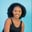Related Tags

python

# How to find the cumulative sum of a 2D array in PythonMaria Elijah

## Overview

Python allows us to perform the cumulative sum of array elements using the cumsum() method from the NumPy library. The numpy.cumsum() method returns the cumulative sum of elements in a given input array over a specified axis.

We can create a two-dimensional (2D) array using a list of lists in Python.

### Syntax

numpy.cumsum(arr, axis=None, dtype=None, out=None)


### Parameters

• arr: This represents the input data.
• axis: This is an optional parameter. It represents the axes along which the operation is to be performed.
• dtype: This is an optional parameter. It represents the return type of the array.
• out: This is an optional parameter. It represents the alternative output array where the result is to be placed.

### Return value

The numpy.cumsum() method returns the cumulative sum of the elements in a given input array over a specified axis.

Note: If the out parameter is specified, it returns an array reference to out.

### Code

The following code shows how to find the cumulative sum of a 2D array using the numpy.cumsum() method.

# Import numpy
import numpy as np
# Create a list
my_list1 = [24,8,3,4,34,8]
my_list2 = [5,7,2,10,15,7]
# Convert to a numpy 2D array
np_list = np.array([my_list1, my_list2])
# Compute and store the cumulative sum of the array elements
np_list_cumsum = np.cumsum(np_list, axis=0)

print(np_list_cumsum)

### Explanation

• Line 2: We import the numpy library.
• Lines 4–5: We create two lists called my_list1 and my_list2.
• Line 7: We convert the lists to a NumPy array that gives us a 2D array. Then, we store the value in a variable called np_list.
• Line 9: We use np.cumsum() to compute the cumulative sum of elements in np_list.
• Line 11: We display the result.

RELATED TAGS

python

CONTRIBUTORMaria Elijah
RELATED COURSES

View all Courses

Keep Exploring

Learn in-demand tech skills in half the time